# Developmental Testing

 Chapter 3: Developmental Testing

 Chapter 3 Developmental Testing

## Contents

Available Software:
RGA

More Resources:
RGA Examples Collection

Reliability growth analysis is the process of collecting, modeling, analyzing and interpreting data from the reliability growth development test program (development testing). In addition, reliability growth models can be applied for data collected from the field (fielded systems). Fielded systems analysis also includes the ability to analyze data of complex repairable systems. Depending on the metric(s) of interest and the data collection method, different models can be utilized (or developed) to analyze the growth processes.

## Example

As an example of such a model development, consider the simple case of a wine glass designed to withstand a fall of three feet onto a level cement surface.

The success/failure result of such a drop is determined by whether or not the glass breaks.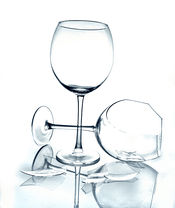Furthermore, assume that:

• You will continue to drop the glass, looking at the results and then adjusting the design after each failure until you are sure that the glass will not break.
• Any redesign effort is either completely successful or it does not change the inherent reliability (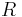$R \,\!$). In other words, the reliability is either 1 or$R \,\!$ ,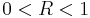$0 .
• When testing the product, if a success is encountered on any given trial, no corrective action or redesign is implemented.
• If the trial fails, then you will redesign the product.
• When the product is redesigned, assume that the probability of fixing the product permanently before the next trial is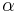$\alpha \,\!$ . In other words, the glass may or may not have been fixed.
• Let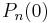$P_{n}(0) \,\!$ and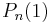$P_{n}(1) \,\!$ be the probabilities that the glass is unreliable and reliable, respectively, just before the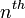$n^{th} \,\!$ trial, and that the glass is in the unreliable state just before the first trial,$P_{1}(0) \,\!$.

Now given the above assumptions, the question of how the glass could be in the unreliable state just before trial$n \,\!$ can be answered in two mutually exclusive ways:

The first possibility is the probability of a successful trial,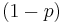$(1-p) \,\!$, where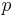$p \,\!$ is the probability of failure in trial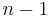$n-1 \,\!$, while being in the unreliable state,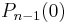$P_{n-1}(0) \,\!$, before the$n-1 \,\!$ trial, or: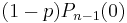$(1-p)P_{n-1}(0)\,\!$

Secondly, the glass could have failed the trial, with probability$p \,\!$, when in the unreliable state,$P_{n-1}(0) \,\!$, and having failed the trial, an unsuccessful attempt was made to fix, with probability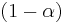$(1-\alpha ) \,\!$, or: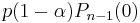$p(1-\alpha)P_{n-1}(0)\,\!$

Therefore, the sum of these two probabilities, or possible events, gives the probability of being unreliable just before trial$n \,\!$ :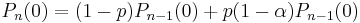$P_{n}(0)=(1-p)P_{n-1}(0)+p(1-\alpha)P_{n-1}(0)\,\!$
or: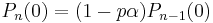$P_{n}(0)=(1-p\alpha)P_{n-1}(0)\,\!$

By induction, since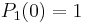$P_{1}(0)=1 \,\!$: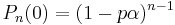$P_{n}(0)=(1-p\alpha)^{n-1}\,\!$

To determine the probability of being in the reliable state just before trial$n \,\!$, the above equation is subtracted from 1, therefore: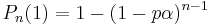$P_{n}(1)=1-(1-p\alpha )^{n-1}\,\!$

Define the reliability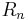$R_{n} \,\!$ of the glass as the probability of not failing at trial$n \,\!$. The probability of not failing at trial$n \,\!$ is the sum of being reliable just before trial$n \,\!$,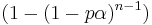$(1-(1-p\alpha)^{n-1}) \,\!$, and being unreliable just before trial$n \,\!$ but not failing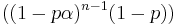$((1-p\alpha )^{n-1}(1-p)) \,\!$, thus: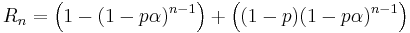$R_{n}=\left (1-(1-p\alpha)^{n-1}\right )+\left ((1-p)(1-p\alpha )^{n-1}\right )\,\!$
or: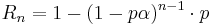$R_{n}=1-(1-p\alpha)^{n-1}\cdot p\,\!$

Now instead of$P_{1}(0)=1 \,\!$, assume that the glass has some initial reliability or that the probability that the glass is in the unreliable state at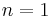$n=1 \,\!$,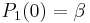$P_{1}(0)=\beta \,\!$, then: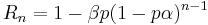$R_{n}=1-\beta p(1-p\alpha )^{n-1}\,\!$

When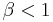$\beta < 1 \,\!$, the reliability at the$n^{th} \,\!$ trial is larger than when it was certain that the device was unreliable at trial$n=1 \,\!$. A trend of reliability growth is observed in the above equation.

Let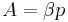$A=\beta p\,\!$ and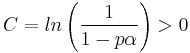$C=ln\left (\frac{1}{1-p\alpha } \right ) > 0 \,\!$, then: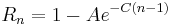$R_{n}=1-Ae^{-C(n-1)}\,\!$

This equation is now a model that can be utilized to obtain the reliability (or probability that the glass will not break) after the$n^{th} \,\!$ trial.

## Applicable Models

The following chapters contain additional information on each of the models that are available for developmental testing: